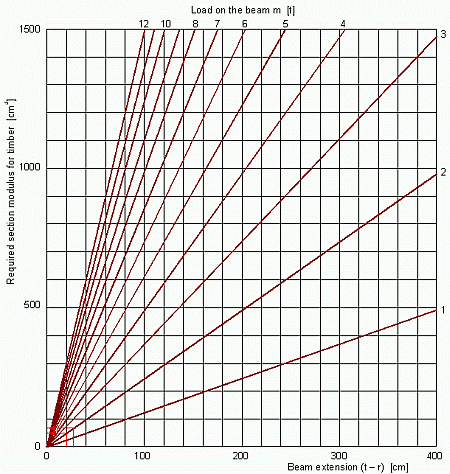6.1.2   Checking the loads to the timber beamsThe coils stowed at the container ends rest on two beams of 10 x 10 cm cross-section and a length of t = 140 cm. The loaded length from the coil is r = 120 cm. With a beam extension of (t - r) = 20 cm and a load of 2 t, the necessary section modulus for each beam is taken from the diagram in Figure 16 with about 50 cm³. The beams have a section modulus of 152 cm³ (Table 1). Also the extension is well within permissible limits. The coils stowed transversely in the middle of the container rest on three longitudinal timbers of 10 x 10 cm cross-section, of which the middle one does not bear due to the deflection of the container bottom. The outer timbers of t = 90 cm are loaded fairly evenly over a length of r = 63 cm. With the extension of (t - r) = 27 cm and the load of 2 t the necessary section modulus for each beam is taken from the diagram in Figure 16 with about 65 cm³. Also this value is less than the existing section modulus of 152 cm³. Also the extension of 27 cm is well within permissible limits.Figure 16: Required section modulus for timber beam beddingContact  |  Site Map  |  Glossary  |  Legal Notice